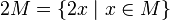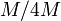# Difference between revisions of "Group cohomology of direct product of Z4 and Z2"

This article gives specific information, namely, group cohomology, about a particular group, namely: direct product of Z4 and Z2.
View group cohomology of particular groups | View other specific information about direct product of Z4 and Z2

## Homology groups for trivial group action

FACTS TO CHECK AGAINST (homology group for trivial group action):
First homology group: first homology group for trivial group action equals tensor product with abelianization
Second homology group: formula for second homology group for trivial group action in terms of Schur multiplier and abelianization|Hopf's formula for Schur multiplier
General: universal coefficients theorem for group homology|homology group for trivial group action commutes with direct product in second coordinate|Kunneth formula for group homology

### Over the integers$H_q(\mathbb{Z}/4\mathbb{Z} \times \mathbb{Z}/2\mathbb{Z};\mathbb{Z}) = \left \lbrace \begin{array}{rl} \mathbb{Z}/4\mathbb{Z} \oplus (\mathbb{Z}/2\mathbb{Z})^{(q+1)/2}, & \qquad 1,3,5,\dots \\ (\mathbb{Z}/2\mathbb{Z})^{q/2}, & \qquad q = 2,4,6,\dots \\\mathbb{Z}, & \qquad q = 0\end{array}\right.$

The first few homology groups are given below:$q$$0$$1$$2$$3$$4$$5$$H_q$$\mathbb{Z}$$\mathbb{Z}/4\mathbb{Z} \oplus \mathbb{Z}/2\mathbb{Z}$$\mathbb{Z}/2\mathbb{Z}$$\mathbb{Z}/4\mathbb{Z} \oplus \mathbb{Z}/2\mathbb{Z} \oplus \mathbb{Z}/2\mathbb{Z}$$\mathbb{Z}/2\mathbb{Z} \oplus \mathbb{Z}/2\mathbb{Z}$$\mathbb{Z}/4\mathbb{Z} \oplus (\mathbb{Z}/2\mathbb{Z})^3$

### Over an abelian group$H_q(\mathbb{Z}/4\mathbb{Z} \times \mathbb{Z}/2\mathbb{Z};M) = \left \lbrace \begin{array}{rl} M/4M \oplus (M/2M)^{(q + 1)/2} \oplus (\operatorname{Ann}_M(2))^{(q - 1)/2}, & \qquad q = 1,3,5,\dots \\ (M/2M)^{q/2} \oplus \operatorname{Ann}_M(4) \oplus (\operatorname{Ann}_M(2))^{q/2}, & \qquad q = 2,4,6,\dots \\ M, & \qquad q = 0 \\\end{array}\right.$

Here,$M/2M$ represents the quotient of$M$ by the subgroup$2M = \{ 2x \mid x \in M \}$,$M/4M$ represents the quotient of$M$ by the subgroup$4M = \{ 4x \mid x \in M \}$,$\operatorname{Ann}_M(2) = \{ x \in M \mid 2x = 0 \}$ and$\operatorname{Ann}_M(4) = \{x \in M \mid 4x = 0 \}$.

## Cohomology groups for trivial group action

FACTS TO CHECK AGAINST (cohomology group for trivial group action):
First cohomology group: first cohomology group for trivial group action is naturally isomorphic to group of homomorphisms
Second cohomology group: formula for second cohomology group for trivial group action in terms of Schur multiplier and abelianization
In general: dual universal coefficients theorem for group cohomology relating cohomology with arbitrary coefficientsto homology with coefficients in the integers. |Cohomology group for trivial group action commutes with direct product in second coordinate | Kunneth formula for group cohomology

### Over the integers$H^q(\mathbb{Z}/4\mathbb{Z} \times \mathbb{Z}/2\mathbb{Z}; \mathbb{Z}) = \left \lbrace \begin{array}{rl} (\mathbb{Z}/2\mathbb{Z})^{(q-1)/2}, & \qquad q = 1,3,5,\dots \\ (\mathbb{Z}/4\mathbb{Z}) \oplus (\mathbb{Z}/2\mathbb{Z})^{q/2}, & \qquad q = 2,4,6,\dots \\ \mathbb{Z}, & \qquad q = 0\end{array}\right.$

### Over an abelian group

Below are the cohomology groups with coefficients in an abelian group$M$:

$Z}/4\mathbb{Z} \times \mathbb{Z}/2\mathbb{Z};M) = \left \lbrace \begin{array}{rl} (M/2M})^{(q-1)/2} \oplus \operatorname{Ann}_M(4) \oplus \operatorname{Ann}_M(2)^{(q+1)/2}, & \qquad q = 1,3,5,\dots \\ (M/4M) \oplus (M/2M)^{q/2} \oplus \operatorname{Ann}_M(2)^{q/2}, & \qquad q = 2,4,6,\dots \\ M, & \qquad q = 0\end{array$

Here,$M/2M$ represents the quotient of$M$ by the subgroup$2M = \{ 2x \mid x \in M \}$,$M/4M$ represents the quotient of$M$ by the subgroup$4M = \{ 4x \mid x \in M \}$,$\operatorname{Ann}_M(2) = \{ x \in M \mid 2x = 0 \}$ and$\operatorname{Ann}_M(4) = \{x \in M \mid 4x = 0 \}$.